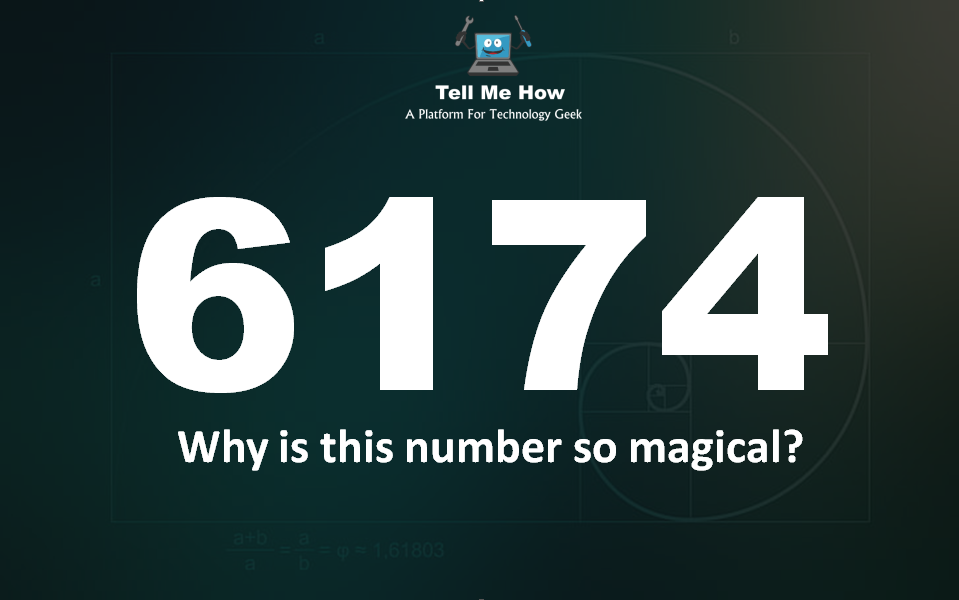## Mind Twister: Why 6174 is magical number?# Why is ‘6174’ discovered by an Indian mathematician called the last magical number?

When you look at the number ‘6174’, for the first time you’ll think that it is an ordinary number. But today I am going to tell you the history of this magical number. From the year 1949 till now, this number has remained a puzzle for mathematicians around the world.

## Who was behind this magical number?

Indian mathematician Dattatreya Ramchandra Kaprekar loved experimenting with numbers. In the process of his experiment, he was introduced to this mysterious number ‘6174’.

Ramchandra Kaprekar, who studied from Mumbai University, spent his life teaching mathematics in a school in Devlali town, Mumbai. He was often called to solve the toughest mathematics questions in schools and colleges.

He used to say that, the way a drunk drinks alcohol to get drunk. It is the same with me in terms of numbers.

## How this magical number being popular?

Actually, during a ‘Mathematics Conference‘ held in Madras in the year 1949, Kaprekar introduced this number to the world. However, many great mathematicians at that time also mocked his discovery. Indian mathematicians even rejected it.

Discussion of this discovery of Kaprekar started not only in India but also in foreign countries. Martin Garder, America’s best-selling author and interested in mathematics until the 1970s, wrote an article about him in a popular science magazine ‘Scientific America‘. Today, Caprekar and his discovery are being recognized. Mathematicians from all over the world are doing research on it.

## Why is this number so magical?

To understand this, look at some interesting facts being told.

For example, choose any number of 4 digits from your mind, but keep in mind that no digit is returned again.

For example, take 1234-

Now write them in descending order – 4321

Then write them in ascending order – 1234
Now subtract the smaller number from the larger number – (4321 – 1234) = 3087
Now write down the number found in the result in decreasing order – 8730

Then write the number found in the result in ascending order – 0378
Now subtract the smaller number from the larger number – (8730 – 0378) = 8352
Now write down the number found in the result in decreasing order – 8532

Then write the number found in the result in ascending order – 2358
Now subtract the smaller number from the larger number – (8532 – 2358) = 6174 (magical number)
Now repeat the magic number ‘6174’ like the above process. That is, decrease it after placing it in increasing and decreasing order.

(7641-1467) = 6174 As you can see, after repeating the process the answer is ‘6174’. If you want, you can repeat it even further, but the result will be ‘6174’.

## Video Example#### By Tell Me How

It is a technology blog and admin has excellent experience in programming from 5+ year. You can contact us at ceo.tellmehow@gmail.com

#### 6 Incredible Ways Technology is Transforming the Service